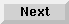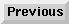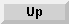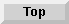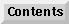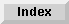3 Optimizing Lisp Programs

# 3.3 Using type-specific operations

To increase the efficiency of compiled code, you should use type-specific operations whenever possible. You will get the most benefit from fixnum arithmetic and hardware-supported floating-point arithmetic.

• Arithmetic that uses values of type`fixnum` is known as fixnum arithmetic. Fixnum values are small integers that are directly represented as machine integers on the underlying hardware. The Compiler can directly code applications of arithmetic operators for which the arguments and the values are known to be of type`fixnum` in the corresponding machine operations; fixnum arithmetic is thus extremely fast. The Common Lisp constants` most-positive-fixnum` and `most-negative-fixnum` define the exact range of fixnum integers for your system.

• Arithmetic operations that use hardware-supported floating-point numbers can be coded in line and are thus extremely fast.

3.3.1 - Using fixnum operations
3.3.2 - Increasing the efficiency of array access
3.3.3 - Propagating type information
3.3.4 - Optimizing code for the MIPS architecture
3.3.5 - Compiling fast floating-point operations

The Advanced User's Guide - 9 SEP 1996Generated with Harlequin WebMaker